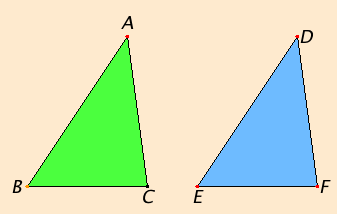# Proposition 4

If two triangles have two sides equal to two sides respectively, and have the angles contained by the equal straight lines equal, then they also have the base equal to the base, the triangle equals the triangle, and the remaining angles equal the remaining angles respectively, namely those opposite the equal sides.

Let ABC and DEF be two triangles having the two sides AB and AC equal to the two sides DE and DF respectively, namely AB equal to DE and AC equal to DF, and the angle BAC equal to the angle EDF.

I say that the base BC also equals the base EF, the triangle ABC equals the triangle DEF, and the remaining angles equal the remaining angles respectively, namely those opposite the equal sides, that is, the angle ABC equals the angle DEF, and the angle ACB equals the angle DFE.If the triangle ABC is superposed on the triangle DEF, and if the point A is placed on the point D and the straight line AB on DE, then the point B also coincides with E, because AB equals DE.

Again, AB coinciding with DE, the straight line AC also coincides with DF, because the angle BAC equals the angle EDF. Hence the point C also coincides with the point F, because AC again equals DF.

C.N.4

But B also coincides with E, hence the base BC coincides with the base EF and equals it.

C.N.4

Thus the whole triangle ABC coincides with the whole triangle DEF and equals it.

And the remaining angles also coincide with the remaining angles and equal them, the angle ABC equals the angle DEF, and the angle ACB equals the angle DFE.

Therefore if two triangles have two sides equal to two sides respectively, and have the angles contained by the equal straight lines equal, then they also have the base equal to the base, the triangle equals the triangle, and the remaining angles equal the remaining angles respectively, namely those opposite the equal sides.

Q.E.D.

## Guide

This is the first of the congruence propositions for triangles. Euclid did not explicitly use the concept of congruence, although it would have simplified his exposition a bit. The definition of congruence would include the hypotheses and conclusions of this proposition, that is, two triangles ABC and DEF are congruent if angles A, B, and C are equal to angles D, E, and F respectively, and sides AB, BC, and AC are equal to sides DE, EF, and DF respectively, and the triangle ABC equals the triangle DEF (by which is meant that they have the same area). In the books on solid geometry, Euclid uses the phrase “similar and equal” for congruence, but similarity is not defined until Book VI, so that phrase would be out of place in the first part of the Elements.

For more discussion of congruence theorems see the note after proposition I.26, the last of the congruence propositions.

Euclid frequently refers to one side of a triangle as its “base,” leaving the other two named “sides.” Any one of the sides might be chosen as the base, but once chosen, it remains the base for the rest of the discussion. This is simply a linguistic device to save words.

#### The method of superposition

The method of proof used in this proposition is sometimes called “superposition.” It apparently is not a method that Euclid prefers since he so rarely uses it, only here in I.4 and in I.8 and III.24, but not in many other propositions in which he could have used it.

It is not clear what is meant by “superposing a triangle on a triangle” means. It has been variously interpreted as actually moving one triangle to cover the other or as simply associating parts of one triangle with parts of the other. For the two triangles illustrated in the figure, you can actually slide one over the other in a continuous motion within the plane. Note, however, that if one triangle is the mirror image of the other, then any continuous motion would require moving one triangle outside of the plane. But the triangles don’t have to be same plane to begin with, and they often are not in the same plane when this proposition is invoked in the books on solid geometry.

Whatever the intended meaning of superposition may be, there are no postulates to allow any conclusions based on superposition. One possibility is to add postulates based on a group of transformations of space, or if restricted to plane geometry, on a group of transformations of the plane. Charles Dodgson (a.k.a. Lewis Carroll) would have said that using group theory is not appropriate to an elementary exposition of Euclidean geometry. Heath has described a more elementary conservative basis in his commentary on this proposition.

Yet another alternative is to simply take this proposition as a postulate, or part of it as a postulate. For instance, Hilbert in his Foundations of Geometry takes as given that under the hypotheses of this proposition that the remaining angles equal the remaining angles. Then, Hilbert proves that the base equals the base.

#### Use of Proposition 4

Of the various congruence theorems, this one is the most used. This proposition is used frequently in Book I starting with the next two propositions, and it is often used in the rest of the books on geometry, namely, Books II, III, IV, VI, XI, XII, and XIII.

Although the two triangles in this proposition appear to be in the same plane, that is not necessary. In Proposition XI.4 and many others in Book XI this proposition is applied to pairs of triangles in different planes.